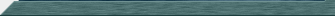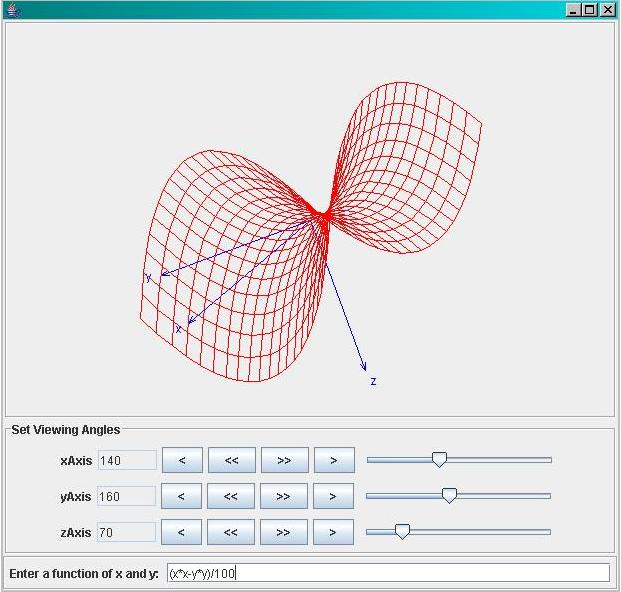exp A mathematical expression evaluation package. Easily allows you to add the ability to evaluate arbitrary mathematical expressions to your java program. Built using the java compiler compiler tool JavaCC Comes with full source code and two example programs. Here's a screen shot of example2:You can spin the 3D image in realtime by moving the three sliders. The gee whiz factor is pretty high :-). To run the example programs (from the directory where you unpacked them) ... Windows run1.bat run2.bat Unix java example1 java example2 The other example is a demonstration of how the exp package can easily be used to provide functionality similar to the unix "bc" utility. Running example1 looks like: ``` C:\EXP> run1 >1+2+3+4+5 15.0 >sin(cos(1/2/3) - pow(exp(2), log(5))) 0.899613861148307 >x = y = 5 5.0 >pow(x, y) 3125.0 ```DownloadReturn to Main